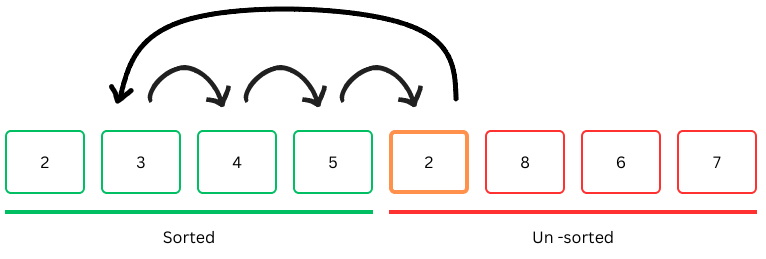back

# Insertion Sort

### Algorithm

Insertion sort works by finding the position of one element at a time in the resulting list and growing it from left to right. At any given moment the list to the left is sorted and the right is unsorted. Repeat the same process for each element in the source list, until the end.

• Works for small sizes
• Not the best for larger sizes``````    public void insertionSort(int[] numbsers) {

for(int i = 1; i < numbsers.length; i++) {

int item = numbsers[i];
int j = i - 1;

while (j >= 0 && item < numbsers[j]) {
numbsers[j + 1] = numbsers[j];
j--;
}

numbsers[j + 1] = item;
}
}

``````